Dr. S. R. Lasker Library Online Catalogue

Normal view

# Applied statistics using stata / Mehmet Mehmetoglu and Tor Georg Jakobsen.

Material type:TextLanguage: English Publication details: 2017. Description: xvi, 356 p. : ill. ; 24 cmISBN: 9781473913233 (pbk); 1473913233; 9781473913226 (hb)DDC classification: 519.50285 Online resources: WorldCat details
Contents:
TOC Research and statistics 1.1 The methodology of statistical research 1.2 The statistical method 1.3 The logic behind statistical inference 1.4 General laws and theories 1.5 Quantitative research papers2. Introduction to Stata 2.1 What is Stata? 2.2 Entering and importing data into Stata 2.3 Data management 2.4 Descriptive statistics and graphs 2.5 Bivariate inferential statistics3. Simple (bivariate) regression 3.1 What is regression analysis? 3.2 Simple linear regression analysis 3.3 Example in Stata4. Multiple regression 4.1 Multiple linear regression analysis 4.2 Example in Stata5. Dummy-Variable Regression 5.1 Why dummy-variable regression? 5.2 Regression with one dummy variable 5.3 Regression with one dummy variable and a covariate 5.4 Regression with more than one dummy variable 5.5 Regression with more than one dummy variable and a covariate 5.6 Regression with two separate sets of dummy variables6. Interaction/moderation effects using regression 6.1 Interaction/moderation effect 6.2 Product-term approach7. Linear regression assumptions and diagnostics 7.1 Correct specification of the model 7.2 Assumptions about residuals 7.3 Influential observations8. Logistic regression 8.1 What is logistic regression? 8.2 Assumptions of logistic regression 8.3 Conditional effects 8.4 Diagnostics 8.5 Multinomial logistic regression 8.6 Ordered logistic regression9. Multilevel analysis 9.1 Multilevel data 9.2 Empty or intercept-only model 9.3 Variance partition / intraclass correlation 9.4 Random intercept model 9.5 Level-2 explanatory variables 9.6 Logistic multilevel model 9.7 Random coefficient (slope) model 9.8 Interaction effects 9.9 Three-level models10. Panel data analysis 10.1 Panel data 10.2 Pooled OLS 10.3 Between effects 10.4 Fixed effects (within estimator) 10.5 Random effects 10.6 Time-series cross-section methods 10.7 Binary dependent variables11. Exploratory factor analysis 11.1 What is factor analysis? 11.2 Factor analysis process 11.3 Composite scores and reliability test 11.4 Example in Stata12. Structural equation modelling and confirmatory factor analysis 12.1 What is structural equation modelling? 12.2 Confirmatory factor analysis 12.3 Latent path analysis13. Critical issues 13.1 Transformation of variables 13.2 Weighting cases 13.3 Robust regression 13.4 Missing data
Summary: Clear, intuitive and written with the social science student in mind, this book represents the ideal combination of statistical theory and practice. It focuses on questions that can be answered using statistics and addresses common themes and problems in a straightforward, easy-to-follow manner. The book carefully combines the conceptual aspects of statistics with detailed technical advice providing both the ‘why’ of statistics and the ‘how’. Built upon a variety of engaging examples from across the social sciences it provides a rich collection of statistical methods and models. Students are encouraged to see the impact of theory whilst simultaneously learning how to manipulate software to meet their needs. --
Tags from this library: No tags from this library for this title.
Star ratingsAverage rating: 0.0 (0 votes)
Holdings
Item type Current library Collection Call number Copy number Status Date due Barcode Item holdsText
Reserve Section
Non-fiction 519.50285 MEA 2017 (Browse shelf(Opens below)) C-1 Not For Loan 28890Text
Circulation Section
Non-fiction 519.50285 MEA 2017 (Browse shelf(Opens below)) C-2 Available 28891
Total holds: 0
##### Browsing EWU Library shelves, Shelving location: Circulation Section Close shelf browser (Hides shelf browser)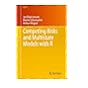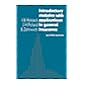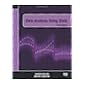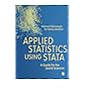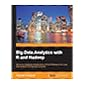519.502 BEC 2012 Competing risks and multistate models with R / 519.5024368 HOI 1999 Introductory statistics with applications in general insurance / 519.50285 KOD 2012 Data analysis using stata / 519.50285 MEA 2017 Applied statistics using stata / 519.50285 PRB 2013 Big data analytics with R and Hadoop / 519.50285 PRB 2013 Big data analytics with R and Hadoop / 519.50285 PRB 2013 Big data analytics with R and Hadoop /

Includes bibliographical references and index

TOC Research and statistics 1.1 The methodology of statistical research 1.2 The statistical method 1.3 The logic behind statistical inference 1.4 General laws and theories 1.5 Quantitative research papers2. Introduction to Stata 2.1 What is Stata? 2.2 Entering and importing data into Stata 2.3 Data management 2.4 Descriptive statistics and graphs 2.5 Bivariate inferential statistics3. Simple (bivariate) regression 3.1 What is regression analysis? 3.2 Simple linear regression analysis 3.3 Example in Stata4. Multiple regression 4.1 Multiple linear regression analysis 4.2 Example in Stata5. Dummy-Variable Regression 5.1 Why dummy-variable regression? 5.2 Regression with one dummy variable 5.3 Regression with one dummy variable and a covariate 5.4 Regression with more than one dummy variable 5.5 Regression with more than one dummy variable and a covariate 5.6 Regression with two separate sets of dummy variables6. Interaction/moderation effects using regression 6.1 Interaction/moderation effect 6.2 Product-term approach7. Linear regression assumptions and diagnostics 7.1 Correct specification of the model 7.2 Assumptions about residuals 7.3 Influential observations8. Logistic regression 8.1 What is logistic regression? 8.2 Assumptions of logistic regression 8.3 Conditional effects 8.4 Diagnostics 8.5 Multinomial logistic regression 8.6 Ordered logistic regression9. Multilevel analysis 9.1 Multilevel data 9.2 Empty or intercept-only model 9.3 Variance partition / intraclass correlation 9.4 Random intercept model 9.5 Level-2 explanatory variables 9.6 Logistic multilevel model 9.7 Random coefficient (slope) model 9.8 Interaction effects 9.9 Three-level models10. Panel data analysis 10.1 Panel data 10.2 Pooled OLS 10.3 Between effects 10.4 Fixed effects (within estimator) 10.5 Random effects 10.6 Time-series cross-section methods 10.7 Binary dependent variables11. Exploratory factor analysis 11.1 What is factor analysis? 11.2 Factor analysis process 11.3 Composite scores and reliability test 11.4 Example in Stata12. Structural equation modelling and confirmatory factor analysis 12.1 What is structural equation modelling? 12.2 Confirmatory factor analysis 12.3 Latent path analysis13. Critical issues 13.1 Transformation of variables 13.2 Weighting cases 13.3 Robust regression 13.4 Missing data

Clear, intuitive and written with the social science student in mind, this book represents the ideal combination of statistical theory and practice. It focuses on questions that can be answered using statistics and addresses common themes and problems in a straightforward, easy-to-follow manner. The book carefully combines the conceptual aspects of statistics with detailed technical advice providing both the ‘why’ of statistics and the ‘how’. Built upon a variety of engaging examples from across the social sciences it provides a rich collection of statistical methods and models. Students are encouraged to see the impact of theory whilst simultaneously learning how to manipulate software to meet their needs. --

Economics AS

Sagar Shahanawaz

There are no comments on this title.